StatLect

We have already seen how boosting works in the training of linear regression models. But that was only a special case (linear model + squared error as loss).

We now describe a generalization called gradient boosting that can be used with arbitrary predictive models and arbitrary loss functions.## Review of general setting

Remember that in general we have a predictive modeland a loss functionthat measures the losses incurred as the predictionis different from the true value.

Our objective is to minimize the risk, or expected lossGradient boosting is a general method used to build sequences of increasingly complex additive models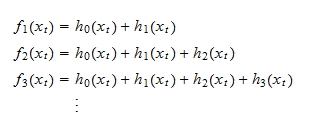whereare very simple models called base learners, andis a starting model (e.g., a model that predicts thatis equal to a constant).

The base learners are trained sequentially: first, thenand so on.

We have already seen this in boosted linear regressions, where the base learners were uni-variate regression models (learning rateregression coefficientinput variable having the highest correlation with the residuals from the previous iteration).

Note that we can also write:which clarifies the fact that each model is obtained by adding a base learner to the previous model.

## Pseudo-residuals

In order to train the base learner, we use the so-called pseudo-residualswhich indicate how we should change our predictions (at the margin) in order to achieve marginal reductions in the loss.

Remark: you can check that pseudo-residuals are scalar multiples of "ordinary" regression residuals when the loss is the squared error: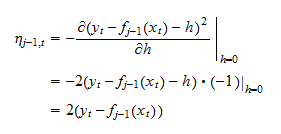Indeed, we used ordinary regression residuals when we trained boosted linear regressions.

## Training base learners

A good base learneris one that takes values close to the pseudo-residuals. In other words,should be close tofor all.

The reason is that:

• a base learner allows us to change our predictions;

• as we explained above, pseudo-residuals tell us how we should change our predictions in order to reduce the loss.

There is no precise rule that tells us how to specify and train our base learners, a task which is largely left to our creativity (even if there are hugely popular methods to do so in specific cases).

However, there are some criteria that are usually followed:

1. the base learners are usually extremely simple models; they are kept simple in order to avoid overfitting as much as possible;

2. the closeness between the values taken by the base learner and the pseudo-residuals is usually measured by the squared errors; in other words, we usually try to minimize3. once we have found a good base learner, we usually apply a learning rateso as to make the increase in overall model complexity more gradual:andWe followed all of these criteria when we trained our first boosted linear regression:

1. our base learners were simple uni-variate regression models;

2. we implicitly trained our base learners by minimizing the squared errors; in fact, we obtained a base learner by running an ordinary least squares regression of the residuals on the chosen regressor;

3. once we found an optimal base learner, we applied a learning rateto it.

## The algorithm

Now, we have all the ingredients that are necessary to build a boosting algorithm, outlined below.

We start from a base function, for example,Then, at each iteration, we perform the following steps:

1. we compute the pseudo-residuals from the previous iteration:2. we find a base learnersuch thatis on average as close as possible to the pseudo-residual(on the training sample);

3. we setwhereis the learning rate (usually);

4. we compute the average loss (empirical risk) of the predictive modelon the validation sample;

5. if the average loss on the validation sample has not been decreasing for a pre-set number of iterations, we stop the algorithm; otherwise, we start the next iteration.

The final boosted model, that we use to make predictions, is the most complex one, produced in the last iteration of the algorithm (boosting round).

## Pseudo-residuals again

Before getting our hands dirty with some examples and Python code, let us compute the pseudo-residuals for some popular loss functions that we have already discussed.

### Squared error

The squared error is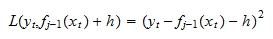and the pseudo-residual, already calculated above, is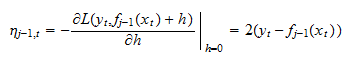### Absolute error

The absolute error isand the pseudo-residual is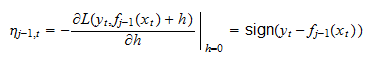where thefunction takes the valueif its argument is weakly positive, and the valueif its argument is strictly negative.

### Log-loss

The log-loss, used in binary classification models, is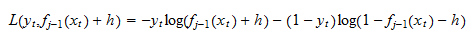and the pseudo-residual isIn practice, when dealing with classification models, we never compute the pseudo-residual in this manner.

What we instead do is to transform the predictions with a logistic function, that is, our prediction ofiswhereso that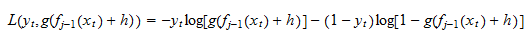and the pseudo-residual, quite magically, turns out to be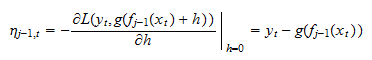## Example: boosted linear regression with absolute error as loss

In this example, we continue to use the same inflation data set used previously.

We are going to slightly modify the code previously used to train a boosted linear regression.

This time, we are going to use the absolute error (AE) as a loss function (instead of the squared error), while everything else will remain unchanged: in particular, our base learners will still be uni-variate linear regressions.

### Import the data and use scikit-learn to split into train-val-test (60-20-20)

We first import the data and split it into train-val-test.

``````# Import the packages used to load and manipulate the data
import numpy as np # Numpy is a Matlab-like package for array manipulation and linear algebra
import pandas as pd # Pandas is a data-analysis and table-manipulation tool

# Import the function that performs sample splits from scikit-learn
from sklearn.model_selection import train_test_split

try:
except:
y = y.values # Transform y into a numpy array

# Print some information about the output variable
print('Class and dimension of output variable:')
print(type(y))
print(y.shape)

# Load the input variables with pandas
try:
except:
x = x.values

# Print some information about the input variables
print('Class and dimension of input variables:')
print(type(x))
print(x.shape)

# Create the training sample
x_train, x_val_test, y_train, y_val_test
= train_test_split(x, y, test_size=0.4, random_state=1)

# Split the remaining observations into validation and test
x_val, x_test, y_val, y_test
= train_test_split(x_val_test, y_val_test, test_size=0.5, random_state=1)

# Print the numerosities of the three samples
print('Numerosities of training, validation and test samples:')
print(x_train.shape, x_val.shape, x_test.shape)``````

The output is:

``````Class and dimension of output variable:
class 'numpy.ndarray'
(270, 1)
Class and dimension of input variables:
class 'numpy.ndarray'
(270, 113)
Numerosities of training, validation and test samples:
162 54 54``````

### Modify the previously created boosted linear regression class

We modify the previously created class for training boosted linear regression models.

We need to modify both the empirical loss and the pseudo-residuals.

The four necessary modifications are highlighted in the comments to the code.

``````# Import package used to make copies of objects
from copy import deepcopy

# Our boosted linear regression (blr) class will implement 3 methods
# (constructor, fit, and predict), as previously seen in sci-kit learn
class blr:
def __init__(self, learning_rate, max_iter, early_stopping):
self.lr = learning_rate
self.max_iter = max_iter
self.early = early_stopping
self.y_mean = 0
self.y_std = 1
self.x_mean = 0
self.x_std = 1
self.theta = 0
self.mses = []

def fit(self, x_train_0, y_train_0, x_val_0, y_val_0):
# Make copies of data to avoid over-writing original dataset
x_train = deepcopy(x_train_0)
y_train = deepcopy(y_train_0)
x_val = deepcopy(x_val_0)
y_val = deepcopy(y_val_0)

# De-mean the output variable
self.y_mean = np.mean(y_train)
y_train -= self.y_mean
y_val -= self.y_mean

# Standardize the output variable
self.y_std = np.std(y_train)
y_train /= self.y_std
y_val /= self.y_std

# De-mean the input variables
self.x_mean = np.mean(x_train, axis=0, keepdims=True)
x_train -= self.x_mean
x_val -= self.x_mean

# Standardize the input variables
self.x_std = np.std(x_train, axis=0, keepdims=True)
x_train /= self.x_std
x_val /= self.x_std

# Initialize counters (total boosting iterations and unproductive iterations)
current_iter = 0
no_improvement = 0

# The starting model has all coefficients equal to zero and predicts a constant zero output
self.theta = np.zeros((x_train.shape, 1))
y_train_pred = 0 * y_train
y_val_pred = 0 * y_val
eta = np.sign(y_train - y_train_pred) # MOD1: we need to change the pseudo-residuals
maes = [np.mean(np.abs(y_val - y_val_pred))] # MOD2: we need to change the empirical risk

# Boosting iterations
while no_improvement < self.early and current_iter < self.max_iter:
current_iter += 1
corr_coeffs = np.mean(x_train * eta, axis=0)
index_best = np.argmax(np.abs(corr_coeffs))
self.theta[index_best] += self.lr * corr_coeffs[index_best]
y_train_pred += self.lr * corr_coeffs[index_best] * x_train[:, [index_best]]
eta = np.sign(y_train - y_train_pred) # MOD3: we need to change the pseudo-residuals
y_val_pred += self.lr * corr_coeffs[index_best] * x_val[:, [index_best]]
maes.append(np.mean(np.abs(y_val - y_val_pred))) # MOD4: we need to change the empirical risk
if maes[-1] > np.min(maes[0:-1]):
no_improvement += 1
else:
no_improvement = 0

# Final output message
print('Boosting stopped after ' + str(current_iter) + ' iterations')

def predict(self, x_test_0):
# Make copies of the data to avoid over-writing original dataset
x_test = deepcopy(x_test_0)

# De-mean input variables using means on training sample
x_test = x_test - self.x_mean

# Standardize output variables using standard deviations on training sample
x_test = x_test / self.x_std

# Return prediction
return self.y_mean + self.y_std * np.dot(x_test,self.theta)``````

### Train the boosted linear regression model

We can now train the boosted regression model with all the 113 input variables.

Note that we change the loss function to mean_absolute_error.

Moreover, we no longer compute the R squared, because it is a metric that is coherent with minimization of the mean squared error and not the mean absolute error (MAE). We instead compute, as a benchmark, the MAE of a naive model that provides a constant prediction, equal to the median ofon the training sample (theorem: with the AE loss, the best constant prediction is the population median).

``````# Import model-evaluation metric from scikit-learn
from sklearn.metrics import mean_absolute_error # MOD5: we change the loss function

# Create a boosted linear regression object
lr = blr(0.1, 10000, 50)

# Train the model
lr.fit(x_train, y_train, x_val, y_val)

# Make predictions on the train, validation and test sets
y_train_pred = lr.predict(x_train)
y_val_pred = lr.predict(x_val)
y_test_pred = lr.predict(x_test)

# Print empirical risk on all sets
print('MAE on training set:')
print(mean_absolute_error(y_train, y_train_pred)) # MOD6: we change the loss function
print('MAE on validation set:')
print(mean_absolute_error(y_val, y_val_pred)) # MOD7: we change the loss function
print('MAE on test set:')
print(mean_absolute_error(y_test, y_test_pred)) # MOD8: we change the loss function
print('MAE of naive model (prediction = median):')
# MOD8: we drop the R squared, but we introduce a new benchmark for comparisons
print(mean_absolute_error(y_test, 0*y_test + np.median(y_train)))
print('')``````

The output is:

``````Boosting stopped after 206 iterations
MAE on training set:
0.10910252442454003
MAE on validation set:
0.21696539910033022
MAE on test set:
0.21493995298974028
MAE of naive model (prediction = median):
0.3151638888888889
``````

### Take-aways from the example

Here are the key take-aways from this example:

• the gradient boosting algorithm is very flexible: with minimal effort, we have been able to change our loss function (from squared error to absolute error);

• we have obtained an algorithm that minimizes the MAE quickly and effectively, despite the large number of input variables; this is an important result because MAE minimization is often desirable (it is robust to outliers and in some cases it makes more sense);

• boosting worked nicely: the empirical losses on the validation and test sets are virtually identical; in other words, we did not overfit on the validation set; moreover, our boosted regression does significantly better than a benchmark model (prediction = median).

Note: the estimation of linear regressions by minimizing the mean absolute error is often called LAD (least absolute deviation) regression. It is a special case of quantile regression (with quantile = 0.5). It is usually performed with linear programming methods that can get quite expensive when the number of variables is as large as in our example.